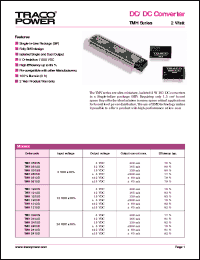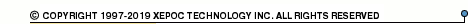More than478 416 registered clientsTMH0505 series datasheets. Manufacturer: TRACO.

 TMH0505D 2 Watt,input voltage range:05V output voltage +/-5V (+/-200mA) DC/DC converter in 6-pin SIP package. Operational temperature range from -40°C to 75°C. Datasheet*) TMH0505S 2 Watt,input voltage range:5V output voltage 5V (400mA) DC/DC converter in 6-pin SIP package. Operational temperature range from -40°C to 75°C. Datasheet*) TMH0512D 2 Watt,input voltage range:5V output voltage +/-12V (+/-80mA) DC/DC converter in 6-pin SIP package. Operational temperature range from -40°C to 75°C. Datasheet*) TMH0512S 2 Watt,input voltage range:5V output voltage 12V (165mA) DC/DC converter in 6-pin SIP package. Operational temperature range from -40°C to 75°C. Datasheet*) TMH0515D 2 Watt,input voltage range:5V output voltage +/-15V (+/-65mA) DC/DC converter in 6-pin SIP package. Operational temperature range from -40°C to 75°C. Datasheet*) TMH0515S 2 Watt,input voltage range:5V output voltage 15V (130mA) DC/DC converter in 6-pin SIP package. Operational temperature range from -40°C to 75°C. Datasheet*) TMH1205D 2 Watt,input voltage range:12V output voltage +/-5V (+/-200mA) DC/DC converter in 6-pin SIP package. Operational temperature range from -40°C to 75°C. Datasheet*) TMH1205S 2 Watt,input voltage range:12V output voltage 5V (400mA) DC/DC converter in 6-pin SIP package. Operational temperature range from -40°C to 75°C. Datasheet*) TMH1212D 2 Watt,input voltage range:12V output voltage +/-12V (+/-80mA) DC/DC converter in 6-pin SIP package. Operational temperature range from -40°C to 75°C. Datasheet*) TMH1212S 2 Watt,input voltage range:12V output voltage 12V (165mA) DC/DC converter in 6-pin SIP package. Operational temperature range from -40°C to 75°C. Datasheet*) TMH1215D 2 Watt,input voltage range:12V output voltage +/-15V (+/-65mA) DC/DC converter in 6-pin SIP package. Operational temperature range from -40°C to 75°C. Datasheet*) TMH1215S 2 Watt,input voltage range:12V output voltage 15V (130mA) DC/DC converter in 6-pin SIP package. Operational temperature range from -40°C to 75°C. Datasheet*) TMH2405D 2 Watt,input voltage range:24V output voltage +/-5V (+/-200mA) DC/DC converter in 6-pin SIP package. Operational temperature range from -40°C to 75°C. Datasheet*) TMH2405S 2 Watt,input voltage range:24V output voltage 5V (400mA) DC/DC converter in 6-pin SIP package. Operational temperature range from -40°C to 75°C. Datasheet*) TMH2412D 2 Watt,input voltage range:24V output voltage +/-12V (+/-80mA) DC/DC converter in 6-pin SIP package. Operational temperature range from -40°C to 75°C. Datasheet*) TMH2412S 2 Watt,input voltage range:24V output voltage 12V (165mA) DC/DC converter in 6-pin SIP package. Operational temperature range from -40°C to 75°C. Datasheet*) TMH2415D 2 Watt,input voltage range:24V output voltage +/-15V (+/-65mA) DC/DC converter in 6-pin SIP package. Operational temperature range from -40°C to 75°C. Datasheet*) TMH2415S 2 Watt,input voltage range:24V output voltage 15V (130mA) DC/DC converter in 6-pin SIP package. Operational temperature range from -40°C to 75°C. Datasheet*)